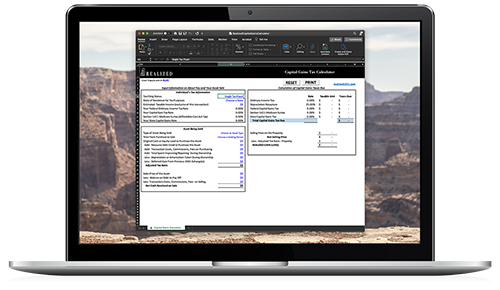Future Value (FV) 2022-06-22 08:00:00

Future value is a time value of money (TVM) concept that represents the expected value, as of a defined date in the future, resulting from compounding present dollar amounts. In its most basic form, the formula for future value (FV) is FV= PV*(1+i)^n, where “PV” equals the present value, “i” represents the interest rate and “n” represents the number of time periods. Future value calculations are useful for investors in estimating the amount of money they will have at future dates based on amounts invested and rates of return achieved today.

For example, if an individual invests \$1,000 today and can earn interest of 6.0% per year, then in 7 years, the investor would have \$1,503.63 (\$1,000 * (1+.06)^7). Like all time value of money calculations, future value equations become more complex as additional variables are added such as future contributions or withdrawals, changing interest or frequency of compounding.

### Cap Gains Calculator For InvestorsEstimate the cap gains tax owed after selling an asset or property

By providing your email and phone number, you are opting to receive communications from Realized. If you receive a text message and choose to stop receiving further messages, reply STOP to immediately unsubscribe. Msg & Data rates may apply. To manage receiving emails from Realized visit the Manage Preferences link in any email received.Capital Gain Tax Rates by State